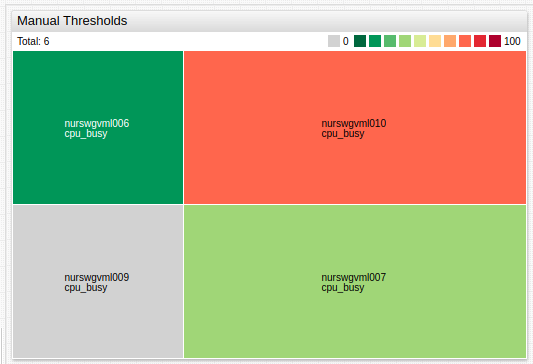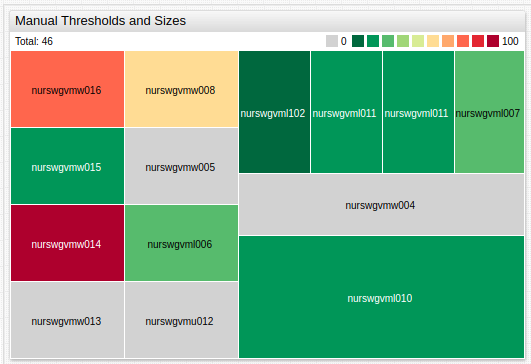# # Treemap Widget

## # Overview

The Treemap Widget displays each series as a colored rectangle according to the magnitude of series value deviation from the threshold.

``````[widget]
type = treemap
entity = nurswgvml0*
colors = green, yellow, red
thresholds = 0, 10, 25, 50

[series]
metric = cpu_busy
``````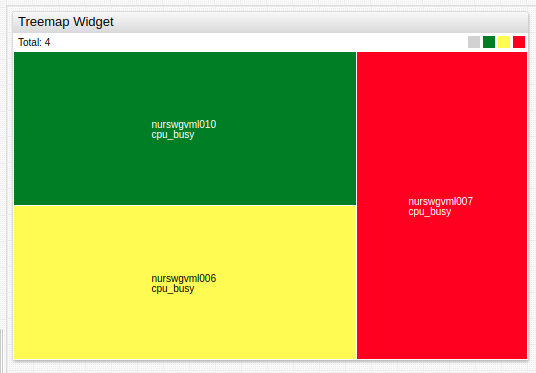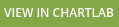## # Widget Settings

• The settings apply to the `[widget]` section.
• Common `[widget]` settings are inherited.
Name Description
`mode` Widget layout mode.
Possible values:
`row` - Align rectangles as rows.
`column` - Align rectangles as columns.
`auto` - Switch between row and column modes depending on widget size.
Example: `mode = row`
`display-total` Display the sum of rectangle sizes in widget header.
Possible values: `false`, `true`.
Default value: `true`.
Example: `display-total = false`
`size-name` Title when `display-total = true`.
Specified `size-name` value is displayed after default title `Total`.
Example: `size-name = Value`
`format-size` `Total` value format.
Refer to Format Settings for possible values.
Example: `format-size = kilobytes`
`font-size` Widget font size.
All text, except widget header is affected.
Default value: `10`.
Example: `font-size = 15`
`color-range` Color palette applied to threshold areas.
Possible values: `red`, `blue`, `black`.
Example: `color-range = red`
`colors` Comma separated list of color names or hex codes, assigned to threshold areas.
The number must be `1` fewer than the number of thresholds.
Example: `colors = green, yellow, red`
Recommended color palette tools: `colorhexa`, `material.io`.
`thresholds` Threshold values.
The number of specified `colors` must be `1` fewer than the number of thresholds.
Example: `thresholds = 0, 25, 50, 75, 100`
`gradient-count` Number of gradients within each threshold rectangle.
Example: `gradient-count = 3`
`total-size` Maximum size of all rectangles combined.
If `total-size` is greater than combined size of all rectangles, additional space is filled `white`.
Example: `total-size = 5`
`palette-ticks` Show legend labels.
Possible values: `false`, `true`.
Default Value: `false`.
Example: `palette-ticks = true`
`rotate-palette-ticks` Rotate legend labels.
Possible values: `false` (horizontal), `true` (vertical).
Default value: `false`.
Example: `rotate-palette-ticks = true`
`range-merge` If `thresholds` is not defined, a different set of ranges is computed for each series based on observed `min` and `max` values within the loaded `timespan`.
`range-merge` computes a single set of ranges for all series in the widget with `min` and `max` for all loaded series.
Possible values: `false`, `true`.
Default value: `false`.
Example: `range-merge = true`

## # Series Settings

• The settings apply to the `[series]` section.
• Common `[series]` settings are inherited.
Name Description
`size` Relative rectangle size.
Possible values: Number, `value`, `value('alias')`.
Default value: `1`.
Examples:
• `size = 3`
• `size = value`

### # Other Settings

• The settings apply to the `[other]` section.
• Specifying the `[other]` section creates an additional rectangle not associated with any series which displays a value or spare capacity.
Name Description
`size` Relative rectangle size.
Possible values: Number, `value`, `value('alias')`.
Default value: `1`.
Examples:
• `size = 3`
• `size = value`
`label` Rectangle label.
Example: `label = Total Capacity`
`color` `[other]` section color.
Default value: `grey`.
Example: `color = black`

### # Properties Settings

• The settings apply to the `[properties]` section.
• In `[series]` or `[other]` section, define properties displayed on mouseover.
• If a value contains `=` or `\`, escape the characters, for example `\=` or `\\`, respectively.
``````[properties]
Data Center = Cuperito
Site = California
Function = SAP DB
``````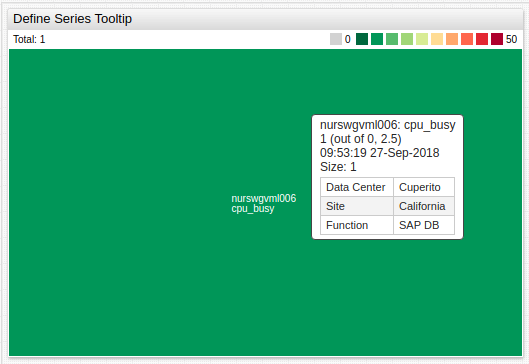## # Examples

### # Manual Thresholds